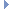chill::QlmAtom Struct Reference

This is the local orientational bond order parameter $$q_{lm}$$, of length $$2l+1$$. More...

#include <bop.hpp>Collaboration diagram for chill::QlmAtom:

## Data Fields

std::vector< YlmAtomptq

## Detailed Description

This is the local orientational bond order parameter $$q_{lm}$$, of length $$2l+1$$.

This complex vector is averaged over the four nearest neighbours, according to the following equation:

$q_{lm}(i) = \frac{1}{N_b(i)} \Sigma_{j=1}^{N_b(i)} Y_{lm}(r_{ij})$

Here, $$N_b(i)=4$$ is the number of nearest neighbours for the molecule $$i$$. This struct contains specifically:

• A complex vector of length $$2l+1$$, calculated according to the equation above

Definition at line 165 of file bop.hpp.

The documentation for this struct was generated from the following file: